×

Search anything:

# Maze game in Python

#### PythonGet this book -> Problems on Array: For Interviews and Competitive Programming

### Contents

1. Maze
2. Rendering the maze
3. Detecting key presses
4. Displaying text
5. Maze generation

In this article, we build a maze game step by step using the Pygame module, conditionals, loops and functions, then we implement random maze generation using depth first search (DFS) algorithm.

## Maze

1. A binary matrix is used to represent a maze. Here we use 0 to represent a valid cell and 1 to represent a wall or a blocked cell.
2. The player starts at upper left corner of the maze and has to reach the bottom right destination using up, down, left and right arrows to move through the maze.
3. Pygame module is used for developing games in Python, to install Pygame:
``````pip install pygame
``````
1. The display module in pygame handles the display window and screen, first we initialize the display module using init() function.
2. Now to display the game window we initialize the maze window using pygame.display.set_mode()
3. Set up the game window dimensions and set the window's caption using set_caption() function.
``````import pygame
import time

pygame.init()

width = 900
height = 650

dark = (109, 104, 117)

gameDisplay = pygame.display.set_mode((width, height))
pygame.display.set_caption("MAZE Game")
``````

## Rendering the maze

1. Create a maze using a matrix representation with 0's representing the maze cells the player can move across and 1's representing the walls or blocked cells.
``````maze=[[1,3,1,0,1,0,1,0,0,0,0,1,1,0,0],
[1,0,1,0,1,0,1,0,1,0,0,1,0,0,0],
[1,0,0,0,1,0,0,0,1,0,0,0,0,1,0],
[1,0,1,0,1,1,0,1,1,1,1,1,0,1,0],
[1,0,1,0,1,0,0,1,0,0,1,0,0,1,0],
[1,1,1,0,1,0,0,0,0,0,1,0,0,1,0],
[1,0,0,0,1,0,0,0,1,0,1,1,1,0,0],
[1,0,1,1,1,1,1,1,1,0,1,0,0,0,0],
[1,0,1,0,0,0,0,0,0,0,1,0,0,0,0],
[1,0,0,0,0,1,1,0,1,1,1,1,1,1,0],
[1,1,1,1,1,1,0,1,0,0,0,0,0,0,2],]
``````
1. Now we need to display the start cell from where the player can start playing, and display the movable maze cells and the walls.
2. We use Pygame module pygame.draw for drawing the maze and specifically the pygame.draw.rect() function to draw rectangles, each rectangle represents a maze cell which can either be a movable cell or a wall.
``````pygame.draw.rect(surface, color, rect)
``````

surface parameter is the surface on which the rectangle is to be drawn.
color parameter takes the color value of the rectangle.
rect parameter takes the coordinate position to draw the rectangle at and also the height and width values of the rectangle.

1. We define a function like renderMaze to render the maze, starting at (0, 0)

Pygame coordinate system: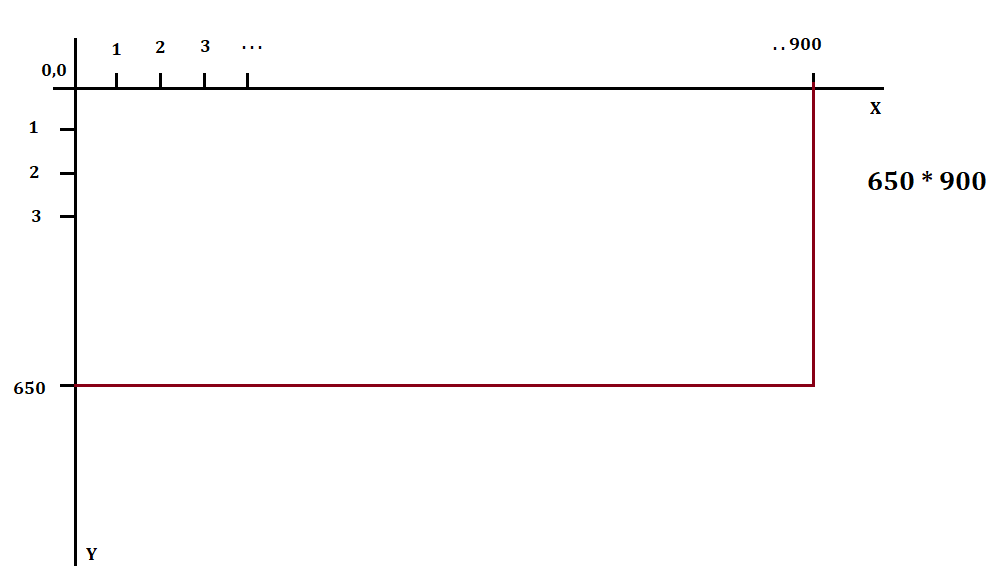1. If the matrix cell value equals 0 then we print a rectangle of one color representing a movable cell in the maze and if the matrix cell equals 1 then we print a rectangle of different color representing a wall in the maze. The destination cell can be represented with a cell value of 2 in the maze matrix.

The player starts at position (1, 0) and the destination is at (11, 15)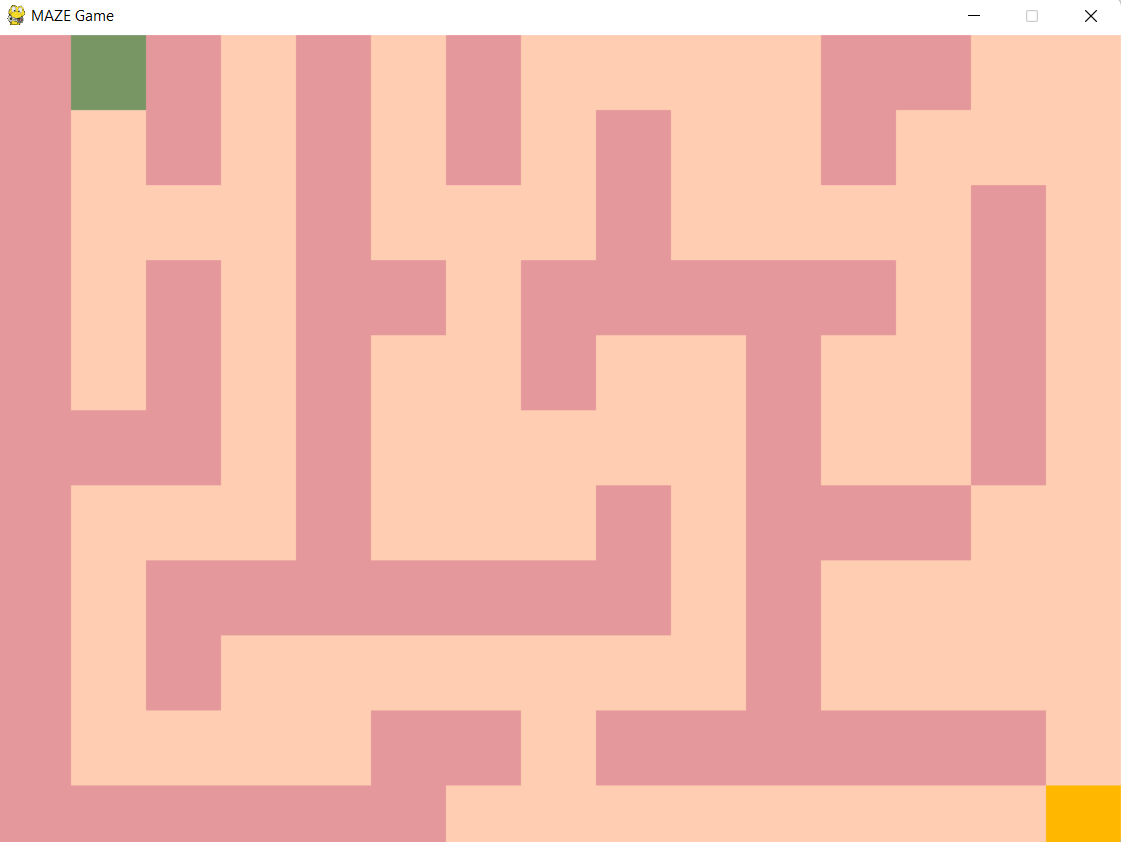``````#function to render the maze
def renderMaze(maze):
x = 0
y = 0
for row in maze:
for block in row:
#block dimension 60*60
# 0 represents movable cell
if block == 0:
pygame.draw.rect(gameDisplay, (255, 205, 178), (x, y, 60, 60))
# 1 represents wall
elif block == 1:
pygame.draw.rect(gameDisplay, (229, 152, 155) ,(x, y, 60, 60))
# 2 represents destination
elif block == 2:
pygame.draw.rect(gameDisplay, (255, 183, 0), (x, y, 60, 60))

# to display the starting cell
elif block == 3:
pygame.draw.rect(gameDisplay, (120, 150, 100), (x, y, 60, 60))

#since dimension of rectangle is 60*60, we move the starting coordinate after each block is drawn
x = x+60
y = y+60
x = 0
``````

Block colors for wall and path: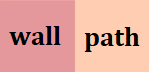## Detecting key presses

1. We need to detect the keyboard input of the player to move in a certain direction in the maze. To detect when a key is pressed, we check if pygame.KEYDOWN type event has occurred.
2. All events are stored in a queue and an event can be accessed using pygame.event.get() function. Every event is an Event object. Each keyboard event has a key attribute associated with it that represents the key pressed on the keyboard.
3. If the player wants to move upwards, downwards or towards left and right, we check if the cell to be reached is a movable cell, a wall or the destination.
4. If the cell to be reached is a movable cell and the key pressed was left arrow or right arrow key then we change the X-coordinate value keeping the Y-coordinate value the same.
5. If the cell to be reached is a movable cell and the key pressed was up arrow or down arrow key then we change the Y-coordinate value keeping the X-coordinate value the same.
6. If the cell to be reached is a wall, then we do nothing and if it's the destination cell then we print that the destination is reached and the game ends.
``````while True:
renderMaze(maze)

#dest determines that destination is reached and ends game.
if dest == 1:
displayText("Yay! Destination reached!")
exit()

#accessing the event queue and the event loop
for event in pygame.event.get():
if event.type == pygame.QUIT:
pygame.quit()

#keydown when a key is pressed on the keyboard
if event.type == pygame.KEYDOWN:

#if left arrow is pressed
if event.key == pygame.K_LEFT:
block = maze[y][x-1]
if block == 0:
maze[y][x-1]= 2
maze[y][x] = 0
x = x-1

elif block == 2:
maze[y][x-1] = 0
maze[y][x] = 0
x = x-1
dest = 1

#if right arrow is pressed
if event.key == pygame.K_RIGHT:
block = maze[y][x +1]

if block == 0:
maze[y][x + 1] =2
maze[y][x]=0
x = x+1
elif block == 2:
maze[y][x+ 1] = 0
maze[y][x] = 0
x = x + 1
dest = 1

#up arrow is pressed
if event.key == pygame.K_UP:
block = maze[y- 1][x]
if block == 0:
maze[y -1][x]= 2
maze[y][x]= 0
y =y -1
elif block == 2:
maze[y -1][x] = 0
maze[y][x] = 0
y = y- 1
dest=1

#down arrow is pressed
if event.key == pygame.K_DOWN:
block=maze[y +1][x]
if block == 0:
maze[y+ 1][x]=2
maze[y][x]=0
y = y + 1
elif block == 2:
maze[y +1][x] = 1
maze[y][x] = 0
y = y+ 1
dest = 1
``````

## Displaying text

1. To display text we use pygame.font module to render fonts and then transfer the text surface onto the game surface we use surface.blit() function.
2. Create a new font object and use pygame.font.Font.render() function to draw text on the surface. Now create a rectangular surface and then surface.blit() function can be used to draw this text on the original game display surface.
``````def displayText(text):
renderFont = pygame.font.Font('freesansbold.ttf', 45)

textsc = renderFont.render(text, True, dark)

surface, rect = textsc, textsc.get_rect()

rect.center = ((width/2),(height/2))

gameDisplay.blit(surface, rect)

pygame.display.update()

#delay of 1 second
time.sleep(1)
``````
1. Once destination is reached, the message is displayed on the window and the game ends.

`displayText("Yay! Destination reached!")`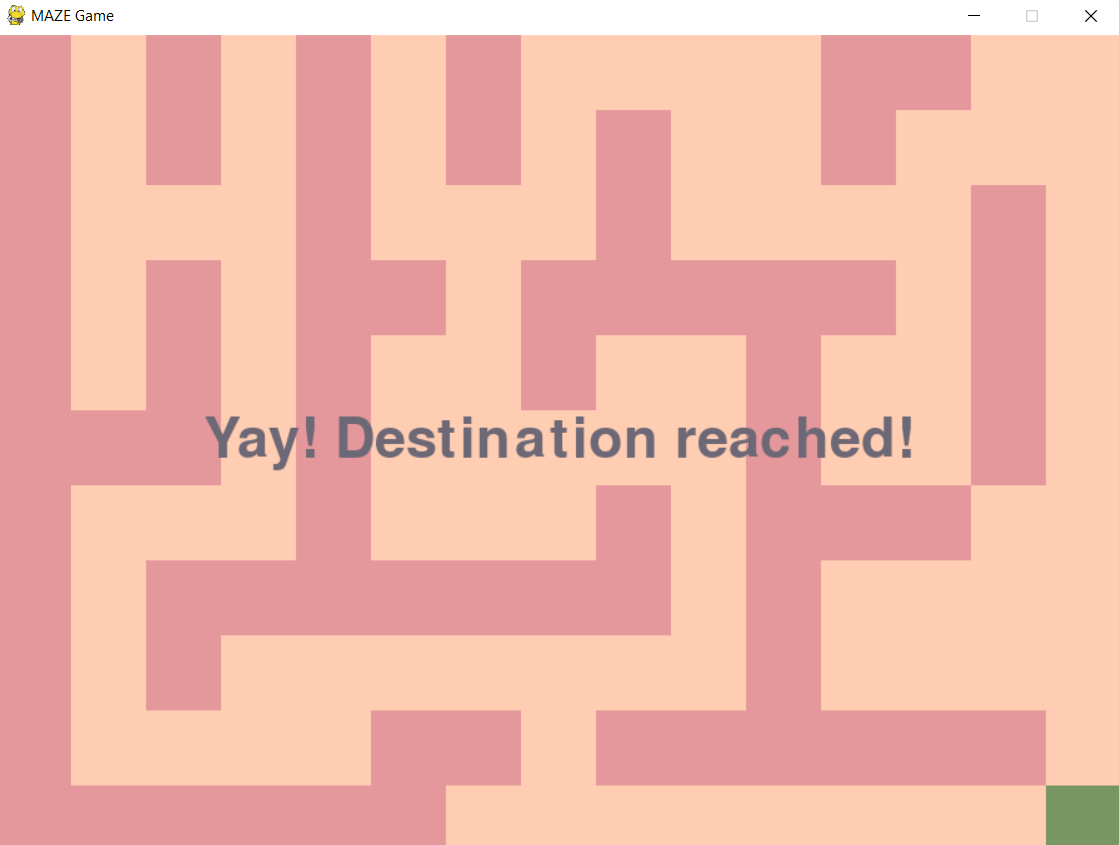## Maze generation

Several maze generation algorithms can be used to generate mazes like Kruskal's algorithm, Depth first search, Prim's algorithm and so on. Here we implement the easiest maze generation algorithm - depth first search.

• Given the height and width of the maze, we create a maze matrix and set all the values to 1. This means that initially there are no paths in the maze and it's filled entirely with walls. '1' represents a wall and '0' represents a path.• Then generate a start point coordinates for the maze randomly using the randint() function.

• Set the start location value in the maze matrix to '0' and we can identify that this location has been visited.

• Now randomly arrange a list of the four directions and iterate through the list - up, down, left and right to carve out a path.

• If the neighbour chosen randomly is already visited or is a dead end then the function backtracks to the previous location which has an unvisited neighbour.

• Algorithm execution finishes when there are no unvisited neighbours of the cells visited.

Algorithm outline:

```dfs(maze, location):
mark current location as visited by marking it as '0' in the maze matrix
choose a direction at random
if there are no unvisited neighbours in that direction then skip
else carve a path from the location to the neighbour chosen at random
perform dfs with the neigbour as the current location - dfs(maze, neighbour)
```
1. Creating a maze matrix given width and height of the maze.
``````maze = [[1 for x in range(width)] for y in range(height)]
``````
1. Generate 2 random numbers to represent the coordinates of the start location of the maze.
``````start_x = random.randint(0, height-1)
``````
1. Set the current location value in the maze matrix to '0' and call the dfs function and pass it the current location coordinates.
``````maze[start_x][start_y] = 0

dfs(maze, height, width, start_x, start_y)
``````
1. Iterate through the directions in the list arranged randomly.
``````import random

directions = [1, 2, 3, 4]
random.shuffle(directions)

for dir in directions:
if dir == 1:
...
elif dir == 2:
...
``````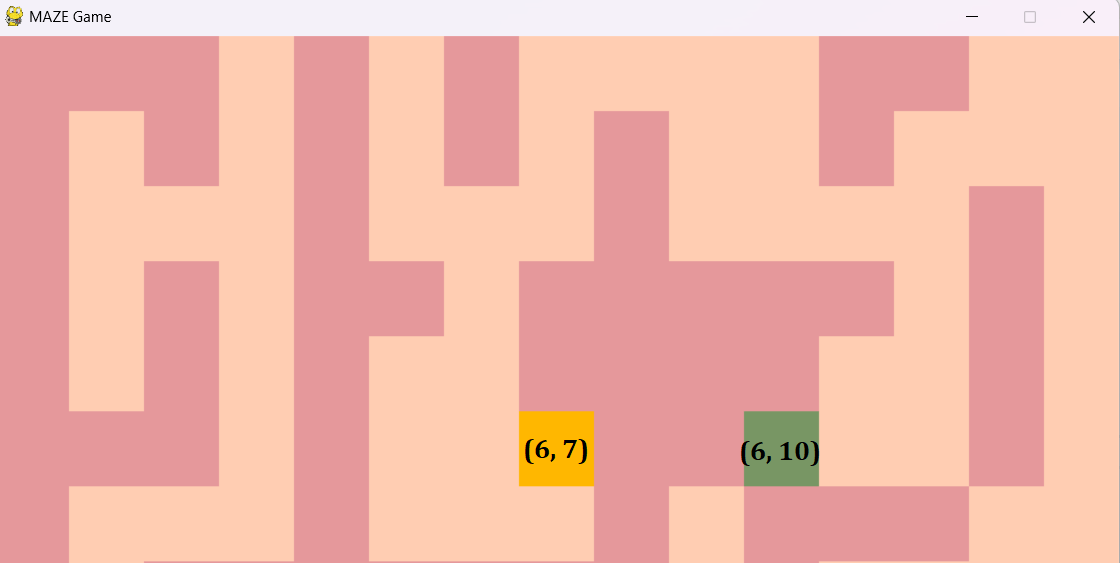• The cell marked in yellow is the current location and assume the direction chosen is right. The maze matrix value, maze = 0 and maze = 1 (unvisited)

• We check if we can move 2 steps to the right by checking if start_y + 2 < width-1.
here height = 7 , width = 15, start_y = 7, start_x = 6.
=> 7(start_y) + 2 = 9
=> 9 < 14 (width-1)

• So now a path can be carved from (6, 7) to (6, 9), maze is changed to 0 from 1 and maze is set to 0.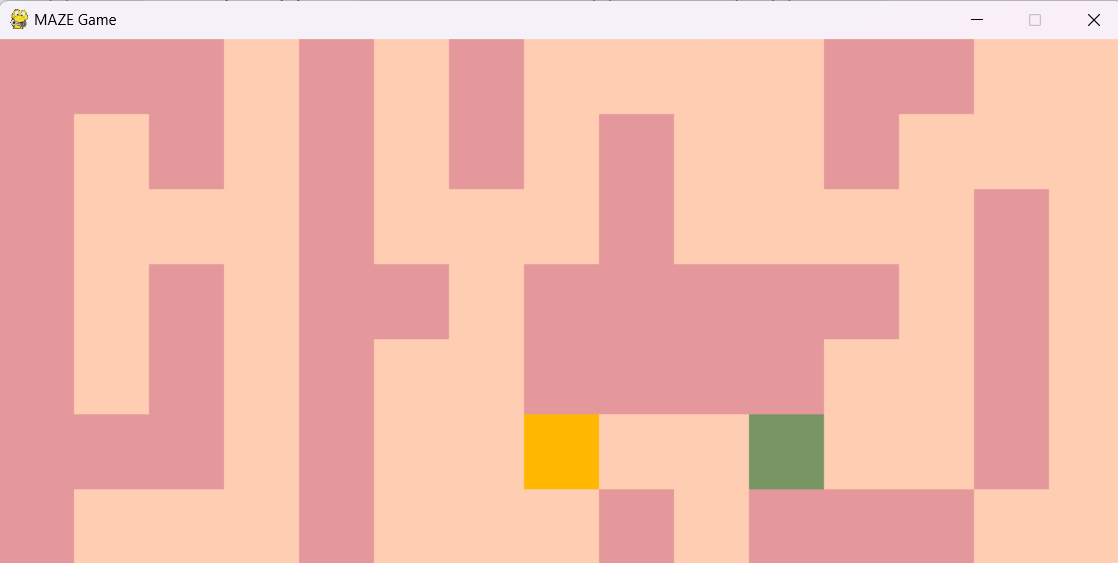• Similarly depending on the direction chosen, we check if a path is possible in that direction and carve a path.

1. Direction - Up
``````if dir == 1:
if (start_x-2) > 0 and maze[start_x - 2][start_y] != 0:
maze[start_x-1][start_y] = 0
maze[start_x-2][start_y] = 0
dfs(maze, height, width, start_x - 2, start_y)
``````
1. Direction - Down
``````elif dir == 2:
if start_x + 2 < height - 1 and maze[start_x + 2][start_y] != 0:
maze[start_x+1][start_y] = 0
maze[start_x+2][start_y] = 0
dfs(maze, height, width, start_x + 2, start_y)
``````
1. Direction - Left
``````elif dir == 3:
if start_y - 2 > 0 and maze[start_x][start_y - 2] != 0:
maze[start_x][start_y - 1] = 0
maze[start_x][start_y - 2] = 0
dfs(maze, height, width, start_x, start_y - 2)
``````
1. Direction - Right
``````elif dir == 4:
if (start_y+2) < width -1 and maze[start_x][start_y + 2] != 0:
maze[start_x][start_y + 1] = 0
maze[start_x][start_y + 2] = 0
dfs(maze, height, width, start_x, start_y + 2)
``````
1. Using the renderMaze function we can print the maze.
Maze with height = 35 and width = 50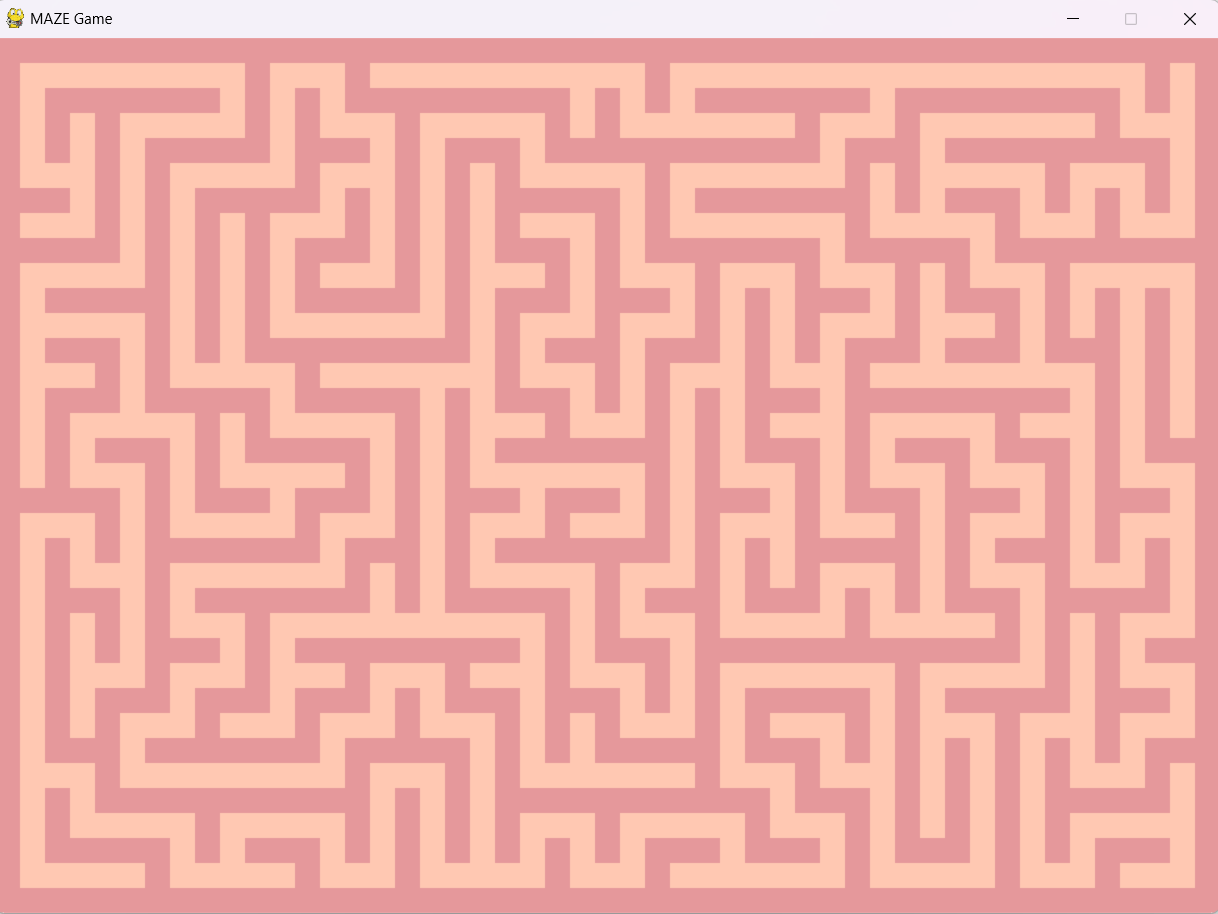Now we can set up a random destination point in the maze which is reachable and the maze game can then be solved!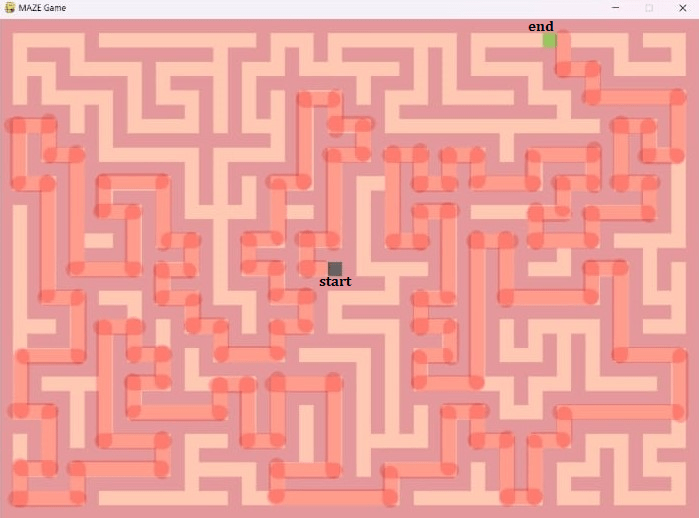In this article at OpenGenus, we've presented a tutorial to build a basic maze game in Python using the Pygame module and also looked at depth first search implementation to generate a maze randomly.#### Sai Siri Chandana Namala

Graduate student of Computer Science at JNTUH College Of Engineering